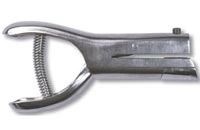MSDSTopics Free Sites FAQ's Regulations Glossary Software Suppliers Books Forum Poll Fun stuff Quiz Store Understand your MSDS with the MS-Demystifier Search ALL our MSDS info

Area Unit ConversionsGet heavy duty hole punches and other fire safety accessories from Safety Emporium.

### Definition

Below is a handy conversion calculator for common (and a few uncommon) units of area. The definition of each term can be found below.

 Area Conversion Calculator(Enter number on the left side; answer appears on the right side) This many: square inchessquare feetsquare yardssquare rodsacresquarter sectionssquare milessquare millimeterssquare centimeterssquare metersareshectaressquare kilometers Equals square inchessquare feetsquare yardssquare rodsacresquarter sectionssquare milessquare millimeterssquare centimeterssquare metersareshectaressquare kilometers

Many of the items you will find on a Safety Data Sheet (SDS) come in both English (U.S. Customary System) and metric (International System or SI or cgs) units. The metric system has been adopted by almost every country except the United States. Even in the U.S., scientists and technical people use the metric system because of its ease of use.

Be sure to see the conversion table for distance/length units if you are not familiar with both systems:

Square Inch
(sq in, in2)
The area of a square measuring one inch on a side. There are 144 square inches in a square foot. 1 sq in = 6.45 sq centimeters.
Square Foot
(sq ft, ft2)
The area of a square measuring one foot on a side. One sq foot = 144 sq inches = 929 sq centimeters.
Square Yard
(sq yd, yd2)
The area of a square measuring one yard on a side. One sq yard = 9 sq feet = 0.836 sq meters.
Square Rod
(sq rd, rd2)
The area of a square measuring one rod (16.5 feet) on a side. One sq rod = 272.5 sq feet = 25.29 sq meters. Uncommon unit.
Acre
(acre)
The area of a square measuring approximately 209 feet on a side. 1 acre = 43,560 square feet = 4047 sq meters = 0.4047 hectares.
Quarter Section
(??)
The area of a square measuring 0.5 mile (2,640 feet) on a side. Four quarter sections make up one section, which is equivalent to one square mile. One quarter section = 160 acres = 0.648 sq kilometers = 6.9 million sq feet.
Square Mile
(sq mi, mi2)
The area of a square measuring one mile (5,280 feet) on a side. Also called a section. One sq mile = 640 acres = 2.59 sq kilometers = 27 million sq feet = 4 quarter sections.
Square Millimeter
(sq mm, mm2)
The area of a square measuring one millimeter (0.001 meters) on a side. One sq mm = 0.01 sq centimeters = 0.00155 sq inches.
Square Centimeter
(sq cm, cm2)
The area of a square measuring one centimeter (0.01 meters) on a side. One sq cm = 100 sq millimeters = 0.155 sq inches.
Square Meter
(sq m, m2)
The area of a square measuring one meter on a side. One sq m = 10.764 sq feet = 1.196 sq yards = 0.0001 ares.
Are
(a)
The area of a square measuring ten meters on a side. One are = 1076 sq feet = 100 sq meters = 0.01 hectares = 0.0247105 acres.
Hectare
(ha)
The area of a square measuring 100 meters on a side. One hectare = 2.47 acres = 10,000 sq meters = 0.01 sq kilometers.
Square Kilometers
(sq km, km2)
The area of a square measuring one kilometer on a side. One sq km = 247 acres = 100 hectares = 10,763,910 sq feet = 0.386 sq miles.

### SDS Relevance

Be very careful to note the units when reading numbers on an SDS. If you ever perform a calculation of any sort, always remember to write the units next to each number in your calculation and make sure that they cancel properly. NASA once lost a \$125 million space probe because of a units error!